Visitors Online: 77 | Wednesday 21st August 2019
 CBSE Guess > Papers > Question Papers > Class XII > 2003 > Physics > Compartment Delhi Set -II PHYSICS—2003 (Set II—Compartment Delhi)
Note: Except for the following questions, all the remaining questions have been already asked in Set I . Q. 2. Write the relation between half-life and decay constant of a radioactive sample. Q. 5. What are the values of (i) the horizontal component of earth's magnetic field and (ii) angle of dip, at the magnetic north pole of the earth? Q. 6. For recharging, n cells, each of emf E, and internal resistance 'r', are connected in series to a resistor 'R' and a d.c. source of e.m.f. V. Deduce the expressions for (i) charging current in the circuit, and (ii) terminal voltage across the cells, during charging. Q. 7. Identify the logic gates marked P and Q in the given logic circuit.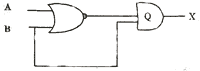Write down the output at X for the inputs (i) A = 0. B = 0 and (ii) A = 1, B = 1. Q. 11. Define the term 'thermoelectric power of a thermocouple'. Draw the curve to show the variation of thermoelectric power with the variation of the temperature of hot junction of the thermocouple keeping cold junction at 00C. Q. 15. What is a rectifier? With the help of a labelled circuit diagram, explain the working of a full wave rectifier. Draw the input and output wave forms. Or, Explain, with the help of a diagram, how (i) depletion layer, and (ii) potential barrier is formed in a p-n junction diode. What happens to the width of depletion layer when the junction is reverse biased? Q. 16. Draw a circuit diagram which can be used to determine the resistance of a given wire. Explain the principle of the experiment and given the formula used. Q. 22. Why is nuclear fusion difficult to carry out? Calculate the energy released in the following nuclear reaction: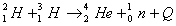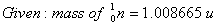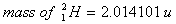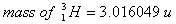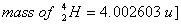Physics 2003 Question Papers Class XII Delhi Outside Delhi Compartment Delhi Compartment Delhi Set I Set I Set I Set I Set II Set II Set II Set II CBSE 2003 Question Papers Class XII